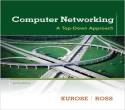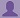SHARE
HELP

To start with, Sr2Jr’s first step is to reduce the expenses related to education. To achieve this goal Sr2Jr organized the textbook’s question and answers. Sr2Jr is community based and need your support to fill the question and answers. The question and answers posted will be available free of cost to all.# Computer Networking : A Top-down Approach

Authors:
James F. Kurose, Keith W. Ross
Exercise:
Problems
Chapter:
Transport Layer
Edition:
6
ISBN:
9780132856201
Question:
3
 Previous Next

###Question

UDP and TCP use 1s complement for their checksums. Suppose you have the following three 8-bit bytes: 01010011, 01100110, 01110100. What is the 1s complement of the sum of these 8-bit bytes? (Note that although UDP and TCP use 16-bit words in computing the checksum, for this problem you are being asked to consider 8-bit sums.) Show all work. Why is it that UDP takes the 1s complement of the sum; that is, why not just use the sum? With the 1s complement scheme, how does the receiver detect errors? Is it possible that a 1-bit error will go undetected? How about a 2-bit error?

###Answer

Given 8-bit bytes are as follows:

01010011

01100110

01110100

Calculate the sum of the given 3 bytes.

 0 1 0 1 0 0 1 1 0 1 1 0 0 1 1 0 1 0 1 1 1 0 0 1

Now add the result with the 3rd byte.

 1 0 1 1 1 0 0 1 0 1 1 1 0 1 0 0 1 0 0 1 0 1 1 0 1

Wrap around the extra bit.

 0 0 1 0 1 1 0 1 1 0 0 1 0 1 1 1 0

The sum three 8-bit bytes is 00101110. Invert all the bits to get the check sum.

Check sum is 11010001.

Now calculate the 1’s compliment of the sum. Convert all 0’s to 1’s and vice versa to find the 1’s compliment.

The 1’s compliment of (sum) 00101110 is 11010001.

It is clear that the 1’s compliment and the checksum are same.

User Datagram Protocol (UDP) uses the 1’s complement as it is same as the checksum of the sum.

The checksum is used by the receiver (host) to identify the errors in the segment.

The process of detecting errors by the receiver:

The receiver performs the following steps at the receiver end to identify the errors in the segment.

• Add all the bytes including checksum.
• Observe the sum.
• If it contains all 1’s then the segment has errors.
• If it contains 1 or more 0’s then the segment contains errors.

Error types that are identified using 1’s compliment method:

• Using 1’s compliment method, it is possible to detect all the 1-bit errors.
• Using 1’s compliment method, there is a possibility that some 2-bit errors are left undetected.

###Ali

• Add all the bytes including checksum.
• Observe the sum.
• If it contains all 1’s then the segment has no errors.
• If it contains 1 or more 0’s then the segment contains errors.

Post the discussion to improve the above solution.